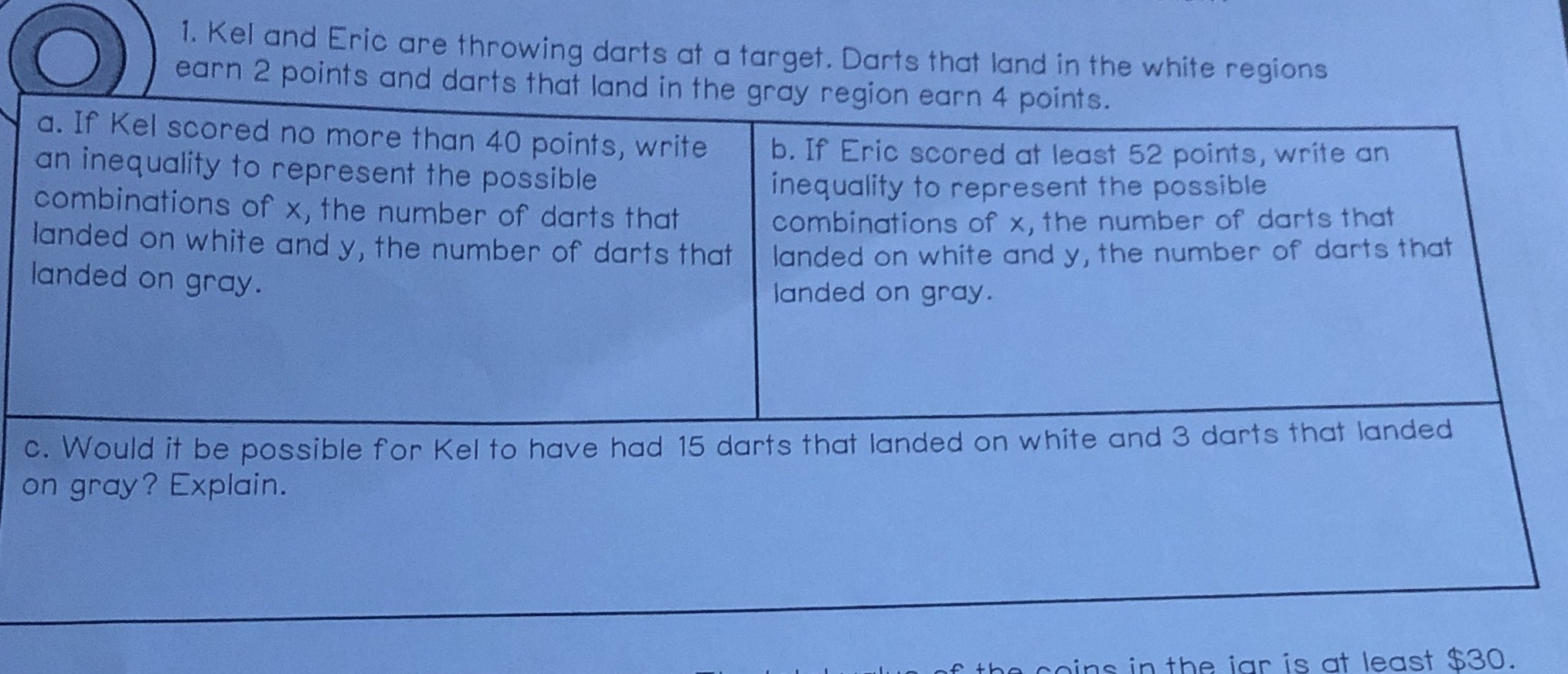### ¿Todavía tienes preguntas de matemáticas?

Pregunte a nuestros tutores expertos
Algebra
Pregunta1. Kel and Eric are throwing darts at a target. Darts that land in the white regions earn $$2$$ points and darts that land in the gray region earn $$4$$ points. a. If Kel scored no more than $$40$$ points, write an inequality to represent the possible combinations of $$x$$ , the number of darts that landed on white and $$y$$ , the number of darts that landed on gray. b. If Eric scored at least $$52$$ points, write an inequality to represent the possible combinations of $$x$$ , the number of darts that landed on white and $$y$$ , the number of darts that landed on gray. c. Would it be possible for Kel to have had $$15$$ darts that landed on white and $$3$$ darts that landed on gray? Explain.

$$x+ 2y\le20\\x+ 2y\le26\\Impossible!\ Because\ 15\times2+ 4\times3= 42> 40$$